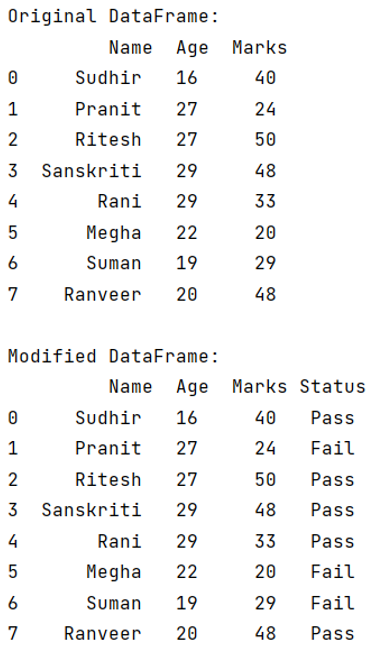# How to insert a given column at a specific position in a Pandas DataFrame?

Given a DataFrame, we have to insert a given column at a specific position. By Pranit Sharma Last updated : September 20, 2023

Columns are the different fields which contains their particular values when we create a DataFrame.

## Problem statement

Given a DataFrame, we have to insert a given column at a specific position.

## Inserting a given column at a specific position in a Pandas DataFrame

For this purpose, we will use pandas.DataFrame.insert() method. This method is used to insert a new column in a DataFrame manually, below is the syntax:

```DataFrame.insert(
loc,
column,
value,
allow_duplicates=False
)

# or
DataFrame.insert(loc='', column='')
```

Parameter(s):

• It takes a parameter loc which means the index where to inert the column.
• It takes another parameter column which is used to assign a name to the new column.
Note

To work with pandas, we need to import pandas package first, below is the syntax:

```import pandas as pd
```

Let us understand with the help of an example.

## Python program to insert a given column at a specific position in a Pandas DataFrame

```# Importing pandas package
import pandas as pd

# Create a dictionary for the DataFrame
dict = {
'Name': ['Sudhir', 'Pranit', 'Ritesh','Sanskriti', 'Rani','Megha','Suman','Ranveer'],
'Age': [16, 27, 27, 29, 29,22,19,20],
'Marks': [40, 24, 50, 48, 33,20,29,48]
}

# Converting Dictionary to Pandas Dataframe
df = pd.DataFrame(dict)

# Display original DataFrame
print("Original DataFrame:\n",df,"\n")

# Creating values for a new column
list = ['Pass','Fail','Pass','Pass','Pass','Fail','Fail','Pass']

# Inserting New column
df.insert(loc=3,column='Status',value=list)

# Display modified DataFrame
print("Modified DataFrame:\n",df)
```

### Output

The output of the above program is: